# Summary of 20 operators commonly used in spark

`val a = sc.parallelize(1 to 9, 3)   # x => *2 is a function, x is the incoming parameter, ie each element of the RDD, x*2 is the return valueval b = a.map(x => x*2)a.collect   # Array[Int] = Array(1, 2, 3, 4, 5, 6, 7, 8, 9)  b.collect   # Array[Int] = Array(2, 4, 6, 8, 10, 12, 14, 16, 18)list/key--->key-valueval a = sc.parallelize(List("dog", "tiger", "lion", "cat", "panther", " eagle"), 2)val b = a.map(x => (x, 1))b.collect.foreach(println(_))# /*# (dog,1)# (tiger,1)# (lion,1)# (cat,1)# (panther,1)# ( eagle,1)# */val l=sc.parallelize(List((1,'a'),(2,'b')))var ll=l.map(x=>(x._1,"PV:"+x._2)).collect()ll.foreach(println)# (1,PVa)# (2,PVb)````
`package testimport scala.Iteratorimport org.apache.spark.SparkConfimport org.apache.spark.SparkContextobject TestRdd {  def sumOfEveryPartition(input: Iterator[Int]): Int = {    var total = 0    input.foreach { elem =>      total += elem    }    total  }  def main(args: Array[String]) {    val conf = new SparkConf().setAppName("Spark Rdd Test")    val spark = new SparkContext(conf)         Val input = spark.parallelize(List(1, 2, 3, 4, 5, 6), 2)//RDD has 6 elements, divided into 2 partitions    val result = input.mapPartitions(             Partition => Iterator(sumOfEveryPartition(partition))) //partition is the passed argument, is a list, and the request is also a list, which is Iterator(sumOfEveryPartition(partition))    result.collect().foreach {      println(_)             # 6 15, partition calculation and    }    spark.stop()  }}`
`val a = sc.parallelize(List("dog", "tiger", "lion", "cat", "panther", " eagle"), 2)val b = a.map(x => (x.length, x))b.mapValues("x" + _ + "x").collect# //Results # Array( # (3,xdogx), # (5,xtigerx), # (4,xlionx), # (3,xcatx), # (7,xpantherx), # (5,xeaglex) # )# val grouped = mds.groupBy(md => md.matched)# grouped.mapValues(x => x.size).foreach(println)`
`val a = sc.parallelize(1 to 4, 2) Val b = a.flatMap(x => 1 to x)//Each element extensionb.collect/* Result Array[Int] = Array( 1,                            1, 2,                            1, 2, 3,                            1, 2, 3, 4)*/`
`val a = sc.parallelize(List((1,2),(3,4),(5,6)))val b = a.flatMapValues(x=>1 to x)b.collect.foreach(println(_))/* result(1,1)(1,2)(3,1)(3,2)(3,3)(3,4)(5,1)(5,2)(5,3)(5,4)(5,5)(5,6)*/val list = List(("mobin",22),("kpop",20),("lufei",23))val rdd = sc.parallelize(list)val mapValuesRDD = rdd.flatMapValues(x => Seq(x,"male"))mapValuesRDD.foreach(println)Output:(mobin,22)(mobin,male)(kpop,20)(kpop,male)(lufei,23)(lufei,male)If it is mapValues ​​will output: [comparison difference](mobin,List(22, male))(kpop,List(20, male))(lufei,List(23, male))`
`val arr = List(("A",3),("A",2),("B",1),("B",3))val rdd = sc.parallelize(arr)val reduceByKeyRDD = rdd.reduceByKey(_ +_)reduceByKeyRDD.foreach(println)sc.stop# (A,5)# (A,4)`
`//omitval arr = List(("A",1),("B",2),("A",2),("B",3))val rdd = sc.parallelize(arr)val groupByKeyRDD = rdd.groupByKey()groupByKeyRDD.foreach(println)sc.stop# (B,CompactBuffer(2, 3))# (A,CompactBuffer(1, 2))# Count the number of array summary elements after the keyscala> groupByKeyRDD.mapValues(x => x.size).foreach(println)# (A,2)# (B,2)`
`/ / Omit scval arr = List(("A",1),("B",2),("A",2),("B",3))val rdd = sc.parallelize(arr)val sortByKeyRDD = rdd.sortByKey()sortByKeyRDD.foreach(println)sc.stop# (A,1)# (A,2)# (B,2)# (B,3)#  word frequencyval rdd = sc.textFile("/home/scipio/README.md")val wordcount = rdd.flatMap(_.split(' ')).map((_,1)).reduceByKey(_+_)val wcsort = wordcount.map(x => (x._2,x._1)).sortByKey(false).map(x => (x._2,x._1))wcsort.saveAsTextFile("/home/scipio/sort.txt")# Ascending order, sortByKey(true)`
`val arr = List(("A", 1), ("B", 2), ("A", 2), ("B", 3))val arr1 = List(("A", "A1"), ("B", "B1"), ("A", "A2"), ("B", "B2"))val rdd1 = sc.parallelize(arr, 3)val rdd2 = sc.parallelize(arr1, 3)val groupByKeyRDD = rdd1.cogroup(rdd2)groupByKeyRDD.foreach(println)sc.stop# (B,(CompactBuffer(2, 3),CompactBuffer(B1, B2)))# (A,(CompactBuffer(1, 2),CompactBuffer(A1, A2)))`
`//omitval arr = List(("A", 1), ("B", 2), ("A", 2), ("B", 3))val arr1 = List(("A", "A1"), ("B", "B1"), ("A", "A2"), ("B", "B2"))val rdd = sc.parallelize(arr, 3)val rdd1 = sc.parallelize(arr1, 3)val groupByKeyRDD = rdd.join(rdd1)groupByKeyRDD.foreach(println)# (B,(2,B1))# (B,(2,B2))# (B,(3,B1))# (B,(3,B2)) # (A,(1,A1))# (A,(1,A2))# (A,(2,A1))# (A,(2,A2`
`//omitval arr = List(("A", 1), ("B", 2), ("A", 2), ("B", 3),("C",1))val arr1 = List(("A", "A1"), ("B", "B1"), ("A", "A2"), ("B", "B2"))val rdd = sc.parallelize(arr, 3)val rdd1 = sc.parallelize(arr1, 3)val leftOutJoinRDD = rdd.leftOuterJoin(rdd1)leftOutJoinRDD .foreach(println)sc.stop# (B,(2,Some(B1)))# (B,(2,Some(B2)))# (B,(3,Some(B1)))# (B,(3,Some(B2)))# (C,(1,None))# (A,(1,Some(A1)))# (A,(1,Some(A2)))# (A,(2,Some(A1)))# (A,(2,Some(A2)))`
`//omitval arr = List(("A", 1), ("B", 2), ("A", 2), ("B", 3))val arr1 = List(("A", "A1"), ("B", "B1"), ("A", "A2"), ("B", "B2"),("C","C1"))val rdd = sc.parallelize(arr, 3)val rdd1 = sc.parallelize(arr1, 3)val rightOutJoinRDD = rdd.rightOuterJoin(rdd1)rightOutJoinRDD.foreach(println)sc.stop# (B,(Some(2),B1))# (B,(Some(2),B2))# (B,(Some(3),B1))# (B,(Some(3),B2))# (C,(None,C1))# (A,(Some(1),A1))# (A,(Some(1),A2))# (A,(Some(2),A1))# (A,(Some(2),A2))`
`var rdd1=sc.parallelize(List((1,"a"),(2,"b"),(3,"c")))# rdd1: org.apache.spark.rdd.RDD[(Int, String)] = ParallelCollectionRDD at parallelize at rdd1.lookup(1)# res34: Seq[String] = WrappedArray(a)`
`val filterRdd = sc.parallelize(List(1,2,3,4,5)).map(_*2).filter(_>5)filterRdd.collect# res5: Array[Int] = Array(6, 8, 10)`
`scala> var rdd1 = sc.makeRDD(1 to 10,2)# rdd1: org.apache.spark.rdd.RDD[Int] = ParallelCollectionRDD at makeRDD at :21 scala> rdd1.collect# res23: Array[Int] = Array(1, 2, 3, 4, 5, 6, 7, 8, 9, 10)`
`scala> var rdd1 = sc.makeRDD(1 to 10,2)# rdd1: org.apache.spark.rdd.RDD[Int] = ParallelCollectionRDD at makeRDD at :21 # scala> rdd1.reduce(_ + _)# res18: Int = 55 scala> var rdd2 = sc.makeRDD(Array(("A",0),("A",2),("B",1),("B",2),("C",1)))# rdd2: org.apache.spark.rdd.RDD[(String, Int)] = ParallelCollectionRDD at makeRDD at :21 #  scala> rdd2.reduce((x,y) => {     |       (x._1 + y._1,x._2 + y._2)     |     })res21: (String, Int) = (CBBAA,6)=================================================================18、count()scala> var rdd1 = sc.makeRDD(Array(("A","1"),("B","2"),("C","3")),2)# rdd1: org.apache.spark.rdd.RDD[(String, String)] = ParallelCollectionRDD at makeRDD at :21 scala> rdd1.count# res15: Long = 3`
`scala> var rdd1 = sc.makeRDD(Array(("A","1"),("B","2"),("C","3")),2)# rdd1: org.apache.spark.rdd.RDD[(String, String)] = ParallelCollectionRDD at makeRDD at :21 scala> rdd1.first# res14: (String, String) = (A,1)`
`scala> val aa=List(1,2,3,"asa")# aa: List[Any] = List(1, 2, 3, asa)scala> aa. map {     |   case i: Int => i + 1     |   case s: String => s.length     | }# res16: List[Int] = List(2, 3, 4, 3)`
`val rdd = sc.parallelize(List(1, 2, 3, 4, 5, 6), 3) // mapPartitions operatorrdd.mapPartitions {             val value = {// outer connection resource map}      iterator => iterator.map(_ * value)} // mapPartitionsWithIndex operatorval partitionIndex = (index: Int, iter: Iterator[Int]) => {    iter.toList.map(item => "index:" + index + ": value: " + item).iterator}rdd.mapPartitionsWithIndex(partitionIndex, true).foreach(println(_))/**index:0: value: 1index:0: value: 2index:1: value: 3index:1: value: 4index:2: value: 5index:2: value: 6*/`
`val rdd = sc.parallelize(List((2, "a b c"), (5, "q w e"), (2, "x y z"), (6, "t y")), 2)rdd.flatMapValues(_.split(" ")).collect()/**Array((2,a), (2,b), (2,c), (5,q), (5,w), (5,e), (2,x), (2,y), (2,z), (6,t), (6,y))*/​​​​​​​`

--

--

--

## More from hivefans

Love podcasts or audiobooks? Learn on the go with our new app.

## How to improve your terminal in 5 easy steps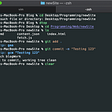## Kubernetes, Docker & Lambda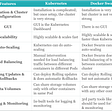## GUILD Token Distribution Overview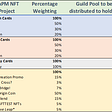## Creating an infinite whiteboard## Why open-source contribution have become more easier in 2020?## Getting Started as a Software Developer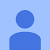## 4 different ways to work with Nebula Graph in Apache Spark## Multiple SparkContext instances error when launching PYSPARK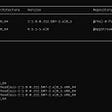## Difference Between External and Internal Tables in Hive (Hands On)## Orchestrating Daily Spark Jobs with EMR in Airflow using AWS Wrangler